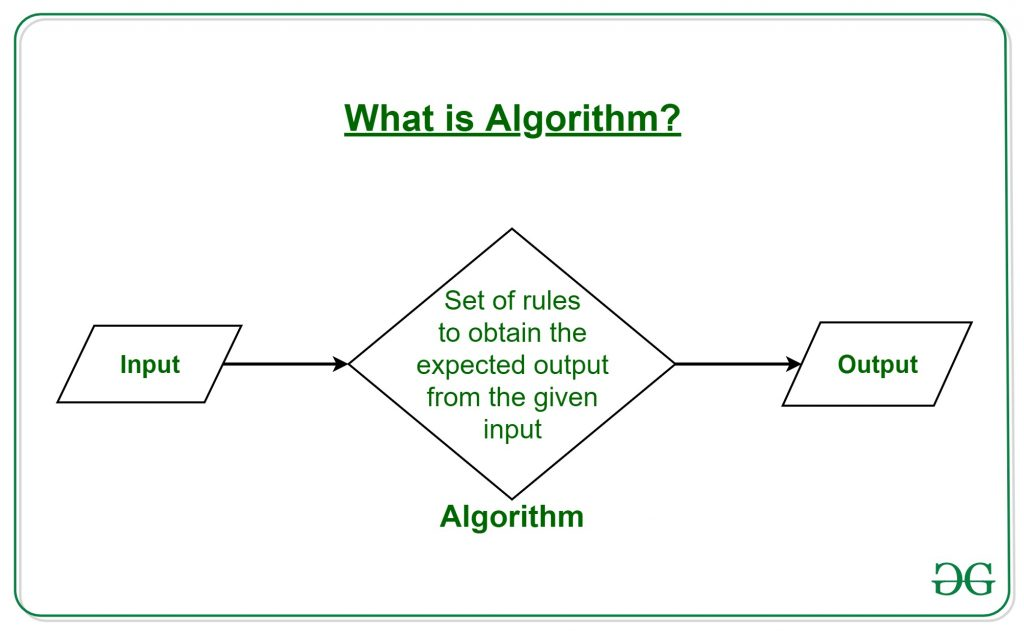# Algorithms

• Last Updated : 10 Aug, 2022

## What is an Algorithm?

The word Algorithm means ” A  set of rules to be followed in calculations or other problem-solving operations ” Or ” A procedure for solving a mathematical problem in a finite number of steps that frequently by recursive operations “.

Therefore Algorithm refers to a sequence of finite steps to solve a particular problem.

Algorithms can be simple and complex depending on what you want to achieve.## Types of Algorithms:

There are several types of algorithms available. Some important algorithms are:

1. Brute Force Algorithm: It is the simplest approach for a problem. A brute force algorithm is the first approach that comes to finding when we see a problem.

2. Recursive Algorithm: A recursive algorithm is based on recursion. In this case, a problem is broken into several sub-parts and called the same function again and again.

3. Backtracking Algorithm: The backtracking algorithm basically builds the solution by searching among all possible solutions. Using this algorithm, we keep on building the solution following criteria. Whenever a solution fails we trace back to the failure point and build on the next solution and continue this process till we find the solution or all possible solutions are looked after.

4. Searching Algorithm: Searching algorithms are the ones that are used for searching elements or groups of elements from a particular data structure. They can be of different types based on their approach or the data structure in which the element should be found.

5. Sorting Algorithm: Sorting is arranging a group of data in a particular manner according to the requirement. The algorithms which help in performing this function are called sorting algorithms. Generally sorting algorithms are used to sort groups of data in an increasing or decreasing manner.

6. Hashing Algorithm: Hashing algorithms work similarly to the searching algorithm. But they contain an index with a key ID. In hashing, a key is assigned to specific data.

7. Divide and Conquer Algorithm: This algorithm breaks a problem into sub-problems, solves a single sub-problem and merges the solutions together to get the final solution. It consists of the following three steps:

• Divide
• Solve
• Combine

8. Greedy Algorithm: In this type of algorithm the solution is built part by part. The solution of the next part is built based on the immediate benefit of the next part. The one solution giving the most benefit will be chosen as the solution for the next part.

9. Dynamic Programming Algorithm: This algorithm uses the concept of using the already found solution to avoid repetitive calculation of the same part of the problem. It divides the problem into smaller overlapping subproblems and solves them.

10. Randomized Algorithm: In the randomized algorithm we use a random number so it gives immediate benefit. The random number helps in deciding the expected outcome.

### Topics :

Analysis of Algorithms:

Searching and Sorting:

Greedy Algorithms:

Dynamic Programming:

Pattern Searching:

Recent Articles on Pattern Searching

Other String Algorithms:

Backtracking:

Divide and Conquer:

Geometric Algorithms:

Mathematical Algorithms:

Bit Algorithms:

Graph Algorithms:

Introduction, DFS and BFS:

Minimum Spanning Tree:

Shortest Paths:

Connectivity:

Hard Problems:

Maximum Flow:

Misc:

Randomized Algorithms:

Recent Articles on Randomized Algorithms

Branch and Bound:

Recent Articles on Branch and Bound

Quizzes on Algorithms:

Misc:

Please see Data Structures and Advanced Data Structures for Graph, Binary Tree, BST and Linked List based algorithms.

### DSA Self Paced

Get DSA’s most popular course at an affordable price, trusted by over 75,000 students! Built by industry experts with years of experience, it provides you with a complete package of video lectures, practice problems, quizzes, discussion forums, and contests. Prepare for SDE interviews with top-tier firms such as Microsoft, Amazon, and Adobe, as well as other top product-based companies. Start Today!

### Live Courses

Get real-time GFG Live Courses from the best in the field to upskill yourself and get into your dream company. You can attend these live classes from any geographical location, and much like an offline programme, you can ask the instructor any questions you have. Check out GeeksforGeeks valuable Live Courses. – System Design Live, Competitive Programming Live, and more!

### Language Foundation Courses[C Programming / C++ / JAVA / Python ]

With the help of pocket friendly GeeksforGeeks Language Foundation Courses – C Programming | Java Foundation | Python Foundation | C++ Foundation. – you can learn any programming language from scratch and master all of its core principles for a strong programming foundation in the simplest way possible. These courses are targeted at complete beginners who want to get started with programming and build their foundations.

My Personal Notes arrow_drop_up